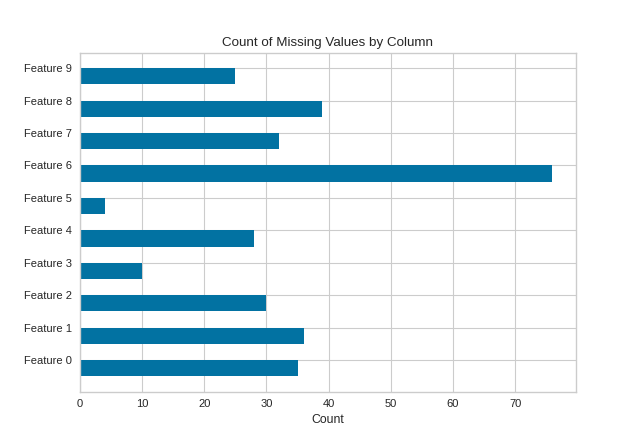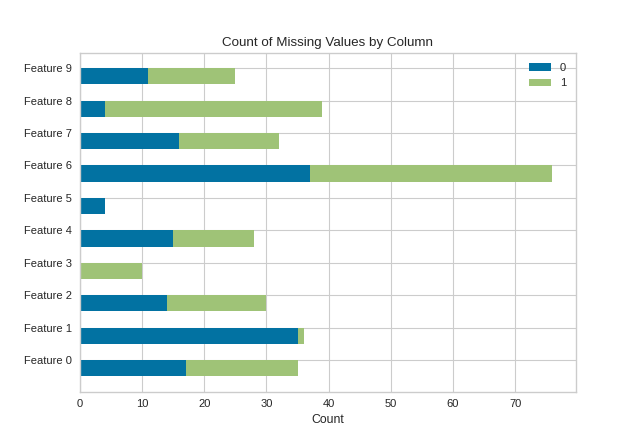# MissingValues Bar¶

The MissingValues Bar visualizer creates a bar graph that counts the number of missing values per feature column. If the target y is supplied to fit, a stacked bar chart is produced.

## Without Targets Supplied¶

import numpy as np

from sklearn.datasets import make_classification
from yellowbrick.contrib.missing import MissingValuesBar

# Make a classification dataset
X, y = make_classification(
n_samples=400, n_features=10, n_informative=2, n_redundant=3,
n_classes=2, n_clusters_per_class=2, random_state=854
)

# Assign NaN values
X[X > 1.5] = np.nan
features = ["Feature {}".format(str(n)) for n in range(10)]

# Instantiate the visualizer
visualizer = MissingValuesBar(features=features)

visualizer.fit(X)        # Fit the data to the visualizer
visualizer.show()        # Finalize and render the figure## With Targets (y) Supplied¶

import numpy as np

from sklearn.datasets import make_classification
from yellowbrick.contrib.missing import MissingValuesBar

# Make a classification dataset
X, y = make_classification(
n_samples=400, n_features=10, n_informative=2, n_redundant=3,
n_classes=2, n_clusters_per_class=2, random_state=854
)

# Assign NaN values
X[X > 1.5] = np.nan
features = ["Feature {}".format(str(n)) for n in range(10)]

# Instantiate the visualizer
visualizer = MissingValuesBar(features=features)

visualizer.fit(X, y=y)        # Supply the targets via y
visualizer.show()             # Finalize and render the figure## API Reference¶

Bar visualizer of missing values by column.

class yellowbrick.contrib.missing.bar.MissingValuesBar(width=0.5, color=None, colors=None, classes=None, **kwargs)[source]

Bases: yellowbrick.contrib.missing.base.MissingDataVisualizer

The MissingValues Bar visualizer creates a bar graph that lists the total count of missing values for each selected feature column.

When y targets are supplied to fit, the output is a stacked bar chart where each color corresponds to the total NaNs for the feature in that column.

Parameters
alphafloat, default: 0.5

A value for bending elments with the background.

markermatplotlib marker, default: |

The marker used for each element coordinate in the plot

colorstring, default: black

The color for drawing the bar chart when the y targets are not passed to fit.

colorslist, default: None

The color palette for drawing a stack bar chart when the y targets are passed to fit.

classeslist, default: None

A list of class names for the legend. If classes is None and a y value is passed to fit then the classes are selected from the target vector.

kwargsdict

Keyword arguments that are passed to the base class and may influence the visualization as defined in other Visualizers.

Examples

>>> from yellowbrick.contrib.missing import MissingValuesBar
>>> visualizer = MissingValuesBar()
>>> visualizer.fit(X, y=y)
>>> visualizer.show()

Attributes
features_np.array

The feature labels ranked according to their importance

classes_np.array

The class labels for each of the target values

draw(X, y, **kwargs)[source]

Called from the fit method, this method generated a horizontal bar plot.

If y is none, then draws a simple horizontal bar chart. If y is not none, then draws a stacked horizontal bar chart for each nan count per target values.

draw_stacked_bar(nan_col_counts)[source]

Draws a horizontal stacked bar chart with different colors for each count of nan values per label.

finalize(**kwargs)[source]

Sets a title and x-axis labels and adds a legend. Also ensures that the y tick values are correctly set to feature names.

Parameters
kwargs: generic keyword arguments.

Notes

Generally this method is called from show and not directly by the user.

get_nan_col_counts(**kwargs)[source]